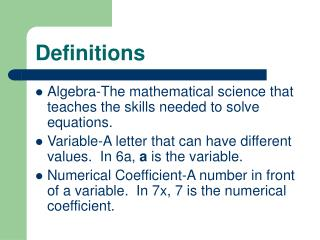DownloadDownload PresentationDefinitions

# Definitions

Download Presentation## Definitions

- - - - - - - - - - - - - - - - - - - - - - - - - - - E N D - - - - - - - - - - - - - - - - - - - - - - - - - - -
##### Presentation Transcript

1. Definitions • Algebra-The mathematical science that teaches the skills needed to solve equations. • Variable-A letter that can have different values. In 6a, a is the variable. • Numerical Coefficient-A number in front of a variable. In 7x, 7 is the numerical coefficient.

2. Exponent-A number to the right of and above a number or variable. In 4x², the 2 is the exponent. • Term-A number, a variable, the product of quotient of numbers and variables. Ex. 3; y; 3x; 2y²z.

3. Algebraic Expression-A mathematical statement which contains a variable. Ex. 4x + 1 • Equation-A mathematical equation containing an equal sign. Ex. 2x – 3 = 7 • Absolute value-The value of a number without any sign. The symbols for absolute value are two vertical bars. Ex. • |-3| = 3

4. The Real Number System • Natural or Counting numbers- 1,2,3,… • Whole Numbers-0,1,2,3,… • Integers-(adds negative numbers)……-2, -1, 0, 1, 2,… • Rational Numbers-(includes fractions) Ex. 4, -3, ¾, .125,… • Irrational Numbers-square roots that are not perfect squares, non-repeating decimals, Ex. .792, /5, .009137306….

5. Properties • Commutative Property of Addition-In addition problems the terms can be moved without changing the value of the problem. 6+2 = 2+6 • Commutative Property of Multiplication-In multiplication problems the terms can be moved without changing the value of the problem. 2·4·3 = 4·3·2

6. Associative Property of Addition-In addition it does not matter which terms you group together first: (6+3) +2 = 6 + (3+2) • Associative Property of Multiplication- In multiplication it does not matter which terms you group together first: (a·b)·c = a·(b·c)

7. Distributive Property-When there is a term next to a parentheses, and no sign between the term and the parentheses, every term inside the parentheses must be multiplied by the term outside the parentheses. 2(3x+7)=2(3x)+2(7) • Additive Inverse-The term you add to another term to get 0. 3a+ (-3a) = 0

8. Multiplicative Inverse-The term you multiply another term by to get a product of 1, also called the reciprocal: 2·½ = 1 • Identity Property of Addition-The addition of zero to any term is equal to the term itself. Ex. 3x + 0= 3x, 27+ 0= 27 • Identity Property of Multiplication-The multiplication of any term by 1 is equal to that term itself. Ex. 1x = x, 1a=5 a=5

9. Equality property: Whatever you do one side of an equation you must do the same thing to the other side. • Examples • Equality Addition Equality Multiplication • 2x –9=+4 2x = 16 • +9 +9 ½·2x = 16 ·½

10. Add and Subtract Positive and Negative Numbers + and + = + and - = - + and - = Subtract the two numbers and take the sign of the larger number

11. Multiplying and Dividing Positive and Negative Numbers +·+ = + +· - = - - · - = +

12. What is the sum of 7 and 4? • What is the difference of 9 and 3? • What is the product of 4 and 2? • What is the quotient of 15 and 3? • What word means add, subtract, multiply, and/or divide?

13. Order of Operation • PEMDAS • Please-parentheses • Excuse-exponents • My-multiplication • Dear-division • Aunt-addition • Sally-subtraction